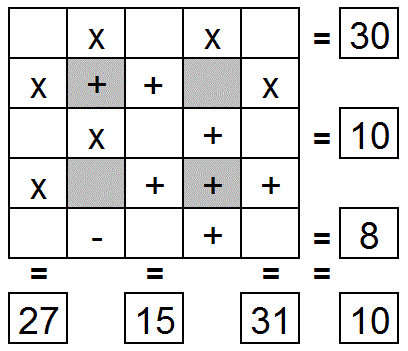Select Page

Each month, a new set of puzzles will be posted.  Come back next month for the solutions and a new set of puzzles, or subscribe to have them sent directly to you.

## MIND-Xpander Logic puzzles

1. The total weight of a tin and cookies it contains is 2 pounds. After ¾ of the cookies are eaten, the tin and the remaining cookies weigh 0.8 pounds. What is the weight of the empty tin in pounds?
2. Insert + &/or – mathematical operation between each digit or group of digits to satisfy the following expression. Note: There is more than one solution.
8 7 6 5 4 3 2 1 = 36
3. What is twice two thirds of three quarters of ten?

## EQUATE+0 puzzle

Each row, column & diagonal is a mathematical expression or equation and you use the numbers 1 to 9 to complete the equations.   Each number can be used only once.  Find the remaining eight numbers that satisfies all the resulting equations. Note – multiplication (x) & division (/) are performed before addition (+) and subtraction (-).## Feedback

There are more than one way of doing these puzzles and may well be more than one answer.  Please let me and others know what alternatives you find by commenting below.  We also welcome general comments on the subject and any feedback you'd like to give.

If you have a question that needs a response from me or you would like to contact me privately, please use the contact form.

## Get more puzzles!

If you've enjoyed doing the puzzles, consider ordering the book; 150+ of the best puzzles in a handy pocket sized format. Click here for full details.

## ROMAN Arithmetic puzzles

Solve the following arithmetic expressions and state their results in Roman Numerals.

1. IV + XCIX = ?,  4 + 99 = 103  (CIII)
2. LIV – XLIV + CLIV = ?,   54 – 44 + 154 = 164 (CLXIV)
3. MV – CCCXC – DLII = ?,   1005 – 390 – 552 = 63  (LXIII)
4. LXXV + CXIX + DCCXVI = ?,   75 + 119 + 716 = 910  (CMX)
5. XI + XXII + XXXIII + XLIV – LV = ?   11 + 22 + 33 + 44 – 55 = 55  (LV)
6. MDCCLXV – MCXX + DXLIV – CDXCVI = ?,   1765 – 1120 + 544 – 496 = 693  (DCXCIII)

## EQUATE+1 puzzle

Each row, column & diagonal is a mathematical expression or equation and you use the numbers 1 to 9 to complete the equations.   Each number can be used only once. ‘One’ number has been provided to get you started. Find the remaining eight numbers that satisfies all the resulting equations. Note – multiplication (x) & division (/) are performed before addition (+) and subtraction (-).